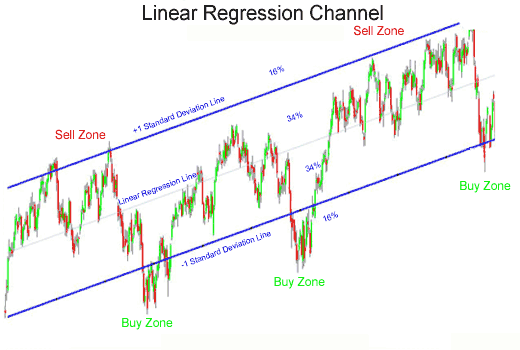## Here is why I consider this market bearish

Look at the chart. I use regression channel. It is a study provided for free in TOS. So the lines of the regression channel shown on the chart are drawn automatically by the study based on the price action of the underlying. It is as automatic as Bollinger Bands. It is expected that the price of the underlying stays within the channel. If it moves out of the channel, the longer it stays out of the channel the the most likely the trend reverses.

This happened in August 2015. The channel was trending up, but after the huge drop it started trending down as seen on the chart. So, just to clarify, those line are not drawn by me manually based on my feeling, but strictly by the study based on the price action and time frame (9 months in this case).

The idea of the Linear Regression Channel 50% is close to the Linear Regression Channel but the upper and lower lines are drawn at the distance of one, not of two, standard deviation from the Linear Regression line. By drawing two parallel lines over and under the Linear Regression line, we obtain a Linear Regression Channel 100%.
At the distance of two standard deviations over and under the Linear Regression line Parallel and equidistant lines are traced. The channel lines are located much farther from the Regression line than any of the closing prices. As far as the Regression channel is a channel for price fluctuations, the top borderline shows resistance whether the bottom channel line shows support. Price values can fall out of the channel for a while, but if the price stays out of the channel for a prolonged period of time, the trend may reverse.
Unlike Linear Regression trend line whose purpose is to show the equilibrium price Linear Regression Channels are the indicators of possible price fluctuations from the trend line.